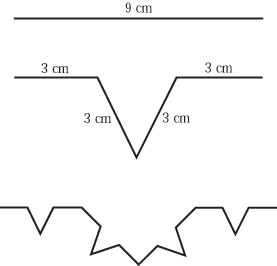HandsOn 8 - Creating Your Own Mathematical Fractal

The mathematical fractal coastline we are about to create is called the "Koch curve.'' You will need:

• a piece of elastic or a rubber band,
• a ruler, and
• a handful of thumb tacks.

In this procedure, we follow the same rule over and over. The central third of a rubber band segment gets turned into a V, like this:Figure 2.9: Beginning the construction of the mathematical fractal called the Koch curve.

1. On a bulletin board, stretch a piece of elastic or a rubber band between two thumb tacks a distance 9 centimeters apart (Figure 2.9). What is the initial length of your straight "coastline?'' If this were a map, a giant could span the entire coast in one step.

2. Place thumb tacks into the elastic 3 centimeters from each end, so that it is divided into 3 equal segments.

3. Take the midpoint of the middle part and pull it perpendicular to the original line to form a V, with each side of the V being 3 centimeters long. Insert a thumb tack to hold it in place. How many segments does your coastline have now - that is, how many straight-line steps does it take to walk exactly along the coastline? What is its total length now? Complete the second row of your copy of Table .

4. There are now four segments, each 3 centimeters long. In each segment, place two thumb tacks so that the segment will be divided into three 1-centimeter parts; then turn the middle part of each segment into a V by pulling it perpendicular to the line and inserting a thumb tack. Each side of each V should be 1 cm. long. How many straight-line steps does it take now to follow the coastline exactly? What is its total length? Add this information to your copy of Table .

5. Now think what the result of the next stage will be. How long will each segment (step) be? How many steps to follow the coastline exactly? What is the total length? Add this information to the copy of Table .

6. Complete the final row of your copy of Table 2.7 by looking at the pattern of other entries.

Table 2.7: Properties of a Koch Curve.
 Length of each step Number of steps to follow coastline Total length (cm.) exactly (cm.) 9 1 9 3 4

 Q2.13: Imagine carrying out the process of turning the middle third of each segment into a V again and again an infinite number of times. How would the curve look? How long would each step be? How many steps would it take to follow the coastline exactly? And what would be the total length of the coastline?

 Q2.14: How does this mathematical fractal differ in appearance from the random fractal coastlines you created in the exercises above?

Plot the table entries on a copy of the log-log graph paper of Figure 2.2. (Note: Recall that you can multiply all entries on both axes by 10 or 1/10 to accommodate the range of numbers to be plotted.) The length of each segment is plotted along the horizontal axis and the number of steps along the vertical axis. Is the result a straight line? If so, what is the slope of this line? The accepted value of the dimension of a Koch curve is 1.26. How does the magnitude of your measured slope compare with this number?

Previous: 2.3 - Mathematical Fractals

Next: 2.4 - Dimensions and Logarithms